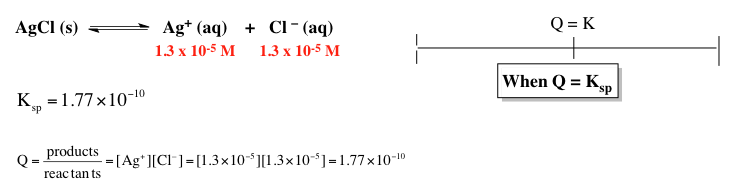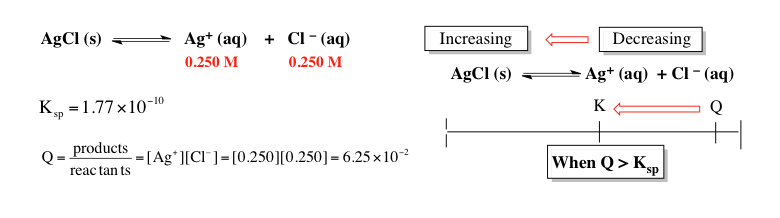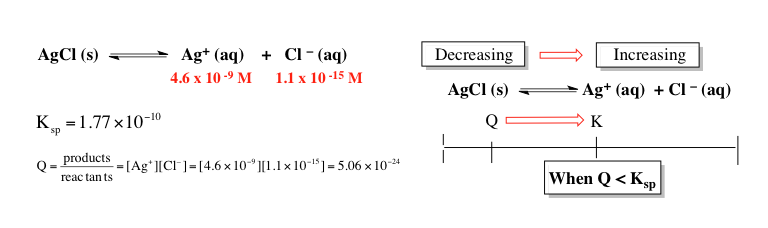Ch.6 - Chemical EquilibriumWorksheetSee all chapters
 Ch.1 - Chemical Measurements 2hrs & 8mins 0% complete Worksheet Ch.2 - Tools of the Trade 1hr & 26mins 0% complete Worksheet Ch.3 - Experimental Error 1hr & 53mins 0% complete Worksheet Ch.4 + 5 - Statistics, Quality Assurance and Calibration Methods 2hrs & 1min 0% complete Worksheet Ch.6 - Chemical Equilibrium 3hrs & 43mins 0% complete Worksheet Ch.7 - Activity and the Systematic Treatment of Equilibrium 1hr & 1min 0% complete Worksheet Ch.8 - Monoprotic Acid-Base Equilibria 1hr & 52mins 0% complete Worksheet Ch.9 - Polyprotic Acid-Base Equilibria 2hrs & 16mins 0% complete Worksheet Ch.10 - Acid-Base Titrations 2hrs & 40mins 0% complete Worksheet Ch.11 - EDTA Titrations 1hr & 37mins 0% complete Worksheet Ch.12 - Advanced Topics in Equilibrium 1hr & 18mins 0% complete Worksheet Ch.13 - Fundamentals of Electrochemistry 2hrs & 27mins 0% complete Worksheet Ch.14 - Electrodes and Potentiometry 43mins 0% complete Worksheet Ch.15 - Redox Titrations 1hr & 14mins 0% complete Worksheet Ch.16 - Electroanalytical Techniques 1hr & 3mins 0% complete Worksheet Ch.17 - Fundamentals of Spectrophotometry 55mins 0% complete Worksheet BONUS: Chemical Kinetics Not available yet

# Solubilty Product Constant

See all sections

The solubility product constant represents the equilibrium value for an ionic solid and its ions in a given solution.

###### Solubility Product Constant

Concept #1: The solubility product constant is a measure of how much an ionic solid will dissolve within a solvent.

Example #1: A hypothetical compound MXhas a molar solubility of 0.00562 M. What is the value of Ksp for MX3?

a) 3.16 x 10-5

b) 2.99 x 10-9

c) 9.48 x 10-5

d) 2.69 x 10-8

Example #2: Which of the following compounds will have the highest molar solubility in pure water?

a) Co(OH)2       Ksp  = 1.3 x 10-15

b) Sr3(PO4)2     Ksp  = 4.0 x 10-28

c) PbCl2           Ksp  =  1.60 x 10-5

d) AgCN          Ksp  =  5.97 x 10-17

e) PbSO4        Ksp  = 1.82 x 10-8

###### Solubility Product Constant Calculations

Example #3: La(OH)3 has a Ksp of 2.0 x 1021. How many grams of La(OH)3 (MW: 189.93 g/mol) are dissolved as hydroxide ions in 2.5 liter of a saturated solution of La(OH)3

Example #4: Find the pH of a saturated solution of Aluminum hydroxide, Al(OH)3. The Ksp of Al(OH)3 is 1.9 x 10-10.

Example #5: Calculate the molar solubility of FeCO3 in a solution of 0.00167 M Na2CO3. Ksp for FeCO3­ is 2.1 x 10-11

Practice: What is the molar solubility of Fe(OH) 3 (s) in a solution that is buffered at pH 3.50 at 25°C? The Ksp of Fe(OH)3 is 6.3 x 10–38 at 25°C.

Practice: The molar solubility of potassium dichromate, K2Cr2O7 (MW: 294.19 g/mol) is 8.3 x 10 -3 g per 100 mL solution at 20°C. Calculate its Ksp

###### The Reaction Quotient & Precipitation

Concept #2: In addition to determining if a chemical reaction is at equilibrium, the reaction quotient can also determine if a precipitate will form.

Concept #3: Comparing the reaction quotient Q to the equilibrium constant K will determine if a precipitate will form or not.

When Q is equal to Ksp the solution is saturated and there is no shifting in the equilibrium position.When Q is greater than Ksp the solution is supersaturated and the reaction shifts in reverse to form more precipitate.When Q is less than Ksp the solution is unsaturated and the reaction shifts forward to form more ions.###### The Reaction Quotient & Precipitation Calculations

Example #6: Will a precipitate form when 0.150 L of 0.100 M Pb(C2H3O2)2 and 0.100 L of 0.20 M NaCl are mixed? The Ksp value of PbCl2 is 1.2 x 10-5

Example #7: What is the minimum pH at which Fe(OH)2 will precipitate if the solution has [Fe2+] = 0.0583 M? Ksp of Fe(OH)2 is 4.87 x 10-17